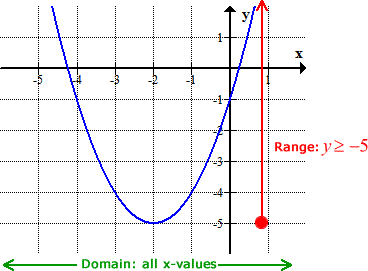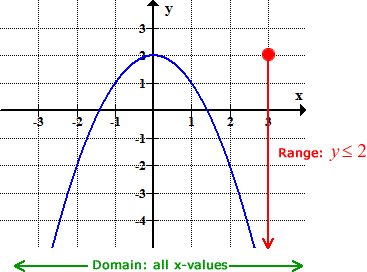# Ctrl N# f(x) means y# GR – Types and Practices

## G.1

GR 1 Graph Relations and Functions , Linear , Quadratic and Exponential

## G.2

GR 2 Graph One Variable Inequality on a Number Line

## G.3

GR 3 Graph two variables Linear Inequality

## G.4

GR

GRS

Graph Inequalities and Ordered Pairs and find if its within the  Solution Set

## G.5

#### GR 2

Graphing a System of Linear Equations

## G.6

#### GR 2

Graphing Quadratic Function and Graph Linear Equation

## G.7

#### GR 2

Graph and Compare Two Linear Equation

GR 1

GR 2

## G.9

#### (x,y)

Graph Relation Trace  Evaluate Function Notation f(x)

## G.10

GRT 2

(x,y)

Graph Relation Trace  Given linear Equations and points ( or table )

## G.11

GRT 3

(x,y)

Graph Relation Trace Given Quadratic Equations and points ( or table )

## G.12

GRT 4

(x,y)

Graph Relation Trace  Evaluate Functions in Real-World Word Problems Given x or y

## G.13

#### GRT 5

Graph Relation Trace Solving Linear Equations

## G.14

GRT 6 Graph Relation Trace  Solving Quadratic Equations

## G.15

GRA 1 Graph Relation Analyze  Find Slope and Zero of Linear Graphs given the Equation

## G.16

GRA 2 Graph Relation Analyze  Solving System of Equations and find intersection

# 2017 STAAR Breakdown Practices

## ⛧ Q 4 – Q 24 GR : Quadratic transformation ⛧

#### – Q 24 : Quadratic transformations. Wider , Narrower.## ⛧ Q 5  Dora (x,y) : Domain and Range of a word problem ⛧

#### – Domain is X which represents the input or the independent and Range is y or the output or the dependent.## ⛧ Q 7 GRT : Function Evaluation from a word problem ⛧

– Evaluate function and find missing variable using Trace Menu 5 1## ⛧ Q 8 – Q 49 KE : Knowledge of Exponential graph attributes ⛧## ⛧ Q 9 – Q 19 LS-C : Causation and Correlation ⛧# Benchmark 1 ( From Question 1 to Question 9 )

## ⛧ Q 14 – Q 46 KQ : Quadratic Graph attributes ( y-int , vertex , max or min , Axis of symmetry ) ⛧## ⛧ Q 15 – Q 31 – Q 35 T : Exponential equations from a word problem ⛧## ⛧ Q 16 GRA : Slope of a line given an equation ⛧ Special## ⛧ Q 17 , Q 28  STO , 5 → Letter : Factoring quadratic expressions using ⛧## ⛧ Q 18  GRA : Solving System equation from a word problem ⛧# Benchmark 2 ( From Question 10 to Question 18 )

## ⛧ Q 20  K-Exponents :  Laws of Exponents ⛧

### – ( multiplication , division , power over power , 0 power , negative power )## ⛧ Q 21   DoRa ( x , y ) : Domain and Range Exponential Graphs and equations ⛧## ⛧ Q 22  GRT : Sequences ⛧## ⛧ Q 23 GRT : Slope-intercept Equation y=mx+b between Two Points ⛧## ⛧ Q 27 Direct Variation ( Cross Multiply ) ⛧## ⛧ Q 29 GR : System of equations given one line and one equation or points ⛧## ⛧ Q 30 DoRa (x,y) : Quadratic Equation ⛧## ⛧ Q 32 – Q 42 KL : Knowledge Linear ( Zero , Y-int , and Slope ) ⛧## ⛧ Q 36 KL : HOY –  VUX   ⛧## ⛧ Q 38 KF : Knowledge Function ⛧## ⛧ Q 44 DoRa (x,y)  : Domain and Range for Line Segments ⛧## ⛧ Q48 GR :Graphing System of Equations ⛧## ⛧ Q 49 GR : Graph Exponential Function ⛧## ⛧ Q 51 STO : Simplify Exponents Expressions ⛧## ⛧ Q 53  DoRa (x,y) : Quadratic Graph ⛧## ⛧ Q 54 GRA : Solving system of equations ⛧